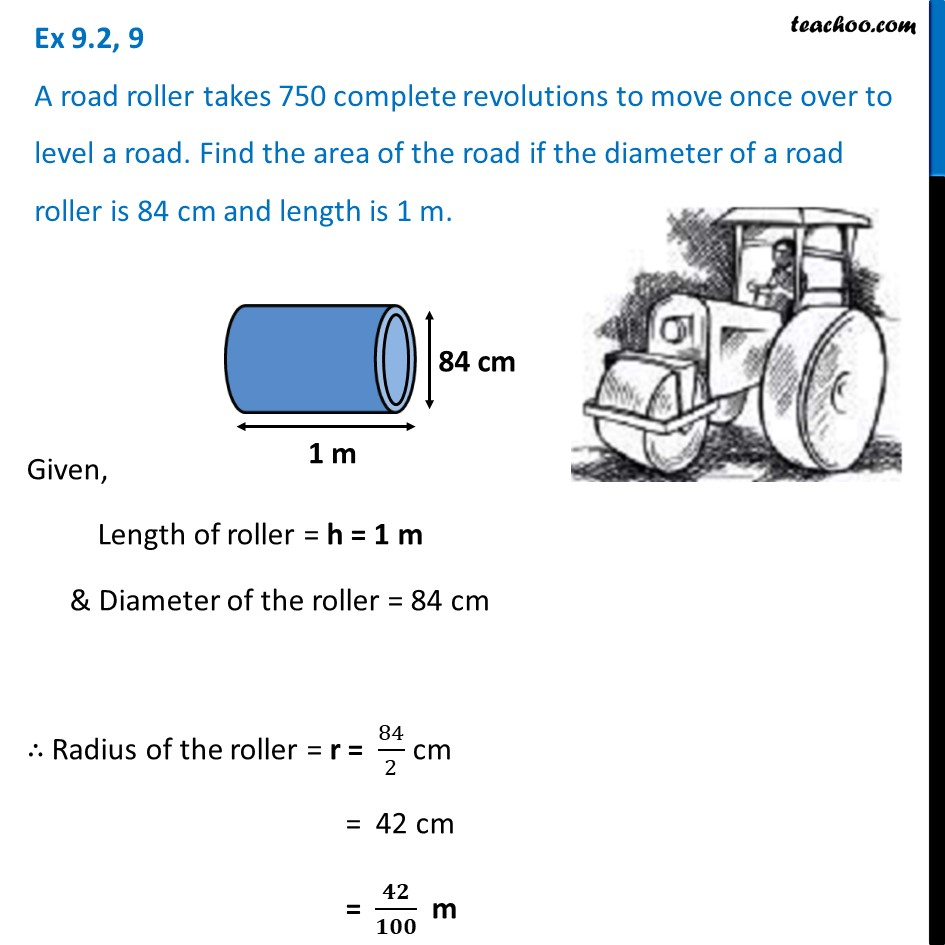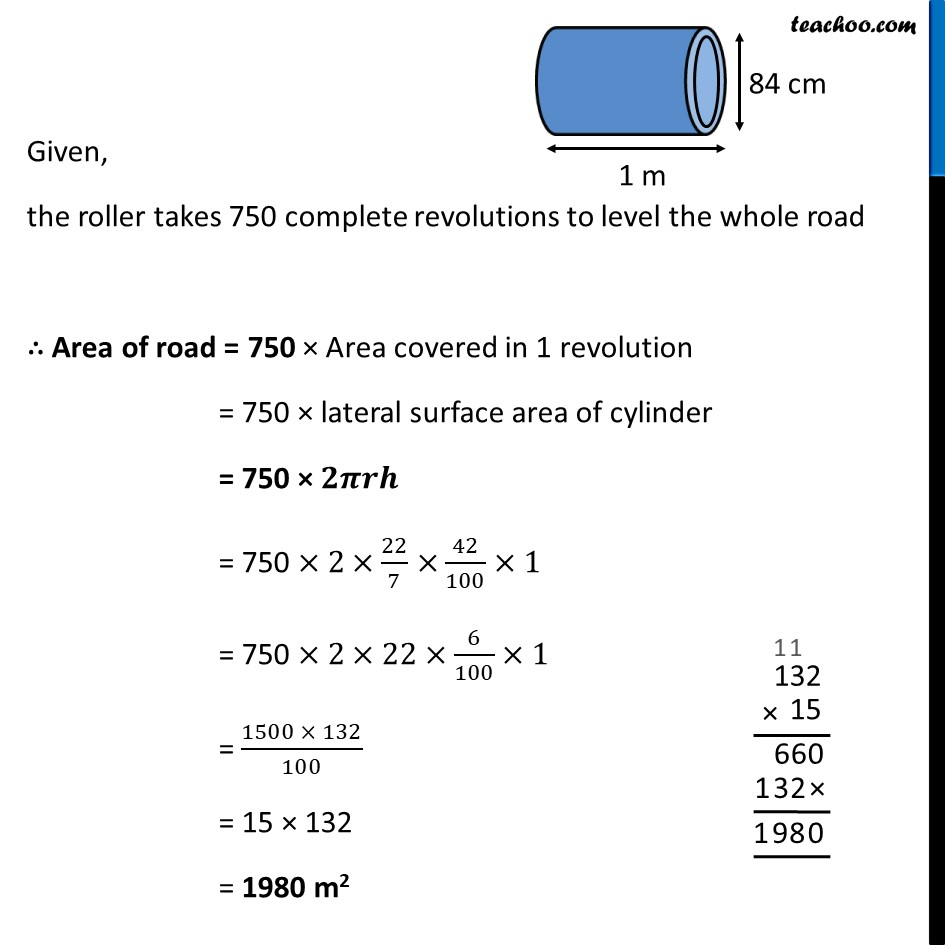Ex 9.2

Chapter 9 Class 8 Mensuration
Serial order wiseLearn in your speed, with individual attention - Teachoo Maths 1-on-1 Class

### Transcript

Ex 9.2, 9 A road roller takes 750 complete revolutions to move once over to level a road. Find the area of the road if the diameter of a road roller is 84 cm and length is 1 m.Given, Length of roller = h = 1 m & Diameter of the roller = 84 cm ∴ Radius of the roller = r = 84/2 cm = 42 cm = 𝟒𝟐/𝟏𝟎𝟎 m Given, the roller takes 750 complete revolutions to level the whole road ∴ Area of road = 750 × Area covered in 1 revolution = 750 × lateral surface area of cylinder = 750 × 𝟐𝝅𝒓𝒉 = 750 ×2×22/7×42/100×1 = 750 ×2×22×6/100×1 = (1500 × 132)/100 = 15 × 132 = 1980 m2